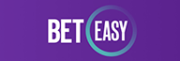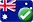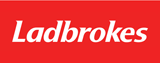# How Are Betting Odds Calculated?

Sports betting is quite a complicated activity. Not only does one have to have a sound knowledge of the particular sport as well as its competitors, but you also have to know exactly how the actual betting side of things work as well. For the more novice punters there are bets which do not involve complicated things such as handicaps, but even in these most basic of wagers betting odds will play a part. Understanding how betting odds work and are calculated is imperative to not only placing a successful and profitable bet, but also to enjoying your entire sports betting experience.

## What Betting Odds Are, Basically

Basically, betting odds are a calculation of the odds or probability of something happening; in this case which team or entrant will win a match or a race. Probability is the basic element which decimal betting odds are derived from, so your first step in understanding them is getting a handle on the whole probability side of things.

The dictionary defines odds as: A representation of the perceived frequency of an event derived from the underlying probability, which enables betting. A coin toss is the best way to demonstrate probability as there are only two potential outcomes; heads or tails. The probability of each one is 0.5, which added together equals the event, or 1. This is worked out using the equation: Favourable outcomes / all possible outcomes. In this case if you had bet that the coin would land on heads, the favourable outcome would be heads; so 0.5 / 1. This is the probability of your bet being successful.

#SiteAURatingBonusBet
15/5100% Match Bonus
Review
24.9/5100% Match up to \$250
Review
34.8/5100% Match

## Applying Probability To Sports Betting

Now that you have a basic idea about probability, you need to be able to apply it to sports betting in order to calculate betting odds. Most sports use betting odds, but the most common one which Australian’s bet on is horse racing. Horse racing uses odds to work out the probability of a runner winning a race, which in turn dictates the amount of money one will win should you bet successfully on that runner.

For example, in a race with four runners, the probability of each wining is 0.25, if they were all even. Bookmakers decide which horse is more likely to win, and increase his odds while decreasing the others. So horse 1 may have a 0.5 chance of winning, while horse 2 has a 0.25 and horse 3 and 4 have 0.125 each. This is where you need to be able to turn probability into decimal odds, which are used by most of the big sports betting sites. Working out you betting odds is done by using the equation 1 / probability of your outcome. So horse 1 would be 1 / 0.5 which gives us 2.0. Horse 3 and 4 would be 1 / 0.125, which would give us 8.0

## How This Affects You

Knowing how to calculate odds directly affects how much money you can make on a bet. In the previous example, horse 1 had a 2:0 chance of winning the race, while horse 4 had an 8:0 of winning. If you bet successfully on horse 1, you will double your money, but if you bet successfully on horse 4, you will get eight times back. Horse 4 therefore is a longer shot, but much more profitable should it win.

Spending some time looking at various odds online in sports such as boxing is a great way to practice calculating betting odds. orse